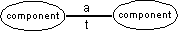﻿ 20-sim webhelp > Modeling Tutorial > Iconic Diagrams > Across and through

# Across and through

A connection between two components transfers energy from one component to the other. This flow of energy can be described in many ways. In 20-sim a uniform approach is chosen:

The flow of energy between two components of an iconic diagram can be characterized by two variables, of which the product is power. These variables are called across (a) and through (t).Across and though as variables of a connection.

The across and though variables make up a combination that is typical for a physical domain. For example voltage and current are used for electrical networks and force and velocity are used for mechanical (translation) systems. The table below shows the variables for the domains that are currently supported in 20-sim.

 Domain across (a) through (t) power across a through t mechanical (translation) velocity v [m/s] force F [N] mechanical (rotation) angular velocity omega [rad/s] torque T [Nm] pneumatic pressure p [Pa] volume flow phi [m3/s] thermal temperature T [K] entropy flow dS [J/Ks] electric voltage u [V] current i [A] hydraulic pressure p [Pa] volume flow phi [m3/s] magnetic magnetomotoric force i [A] flux change df/dt [V] pseudothermal temperature T [K] heat flow dQ [W]

The across and through variables for several domains.

The most general domain is power. Connections of this domain can be used for all domains. For the thermal domain there is one pair of across and through, T and dS, that multiplies to power. The pair T and dQ however is more often used but does not multiply to power. Therefore the domain with these variables is called pseudothermal.

There is a direct relation between the across and through variables of an iconic diagram and the effort and flow variables of a bond graph:

 Domain Non-mechanical domains Mechanical Domains across variable effort flow through variable flow effort

The relation between across and through variables and effort and flow variables.

## Note

 • 20-sim propagates domains. If a connection of the general power domain is tied to a component of the electrical domain, it automatically becomes an electrical connection. The other end of the connection can then only be tied to another component of the electrical domain. In this way it is prevented to connect components of different physical domains.
 • If you want to use a connection of a domain that is not supported or has variables other than across and through, use the general power domain. The across and through variables can then be used as an alias of your own variables.